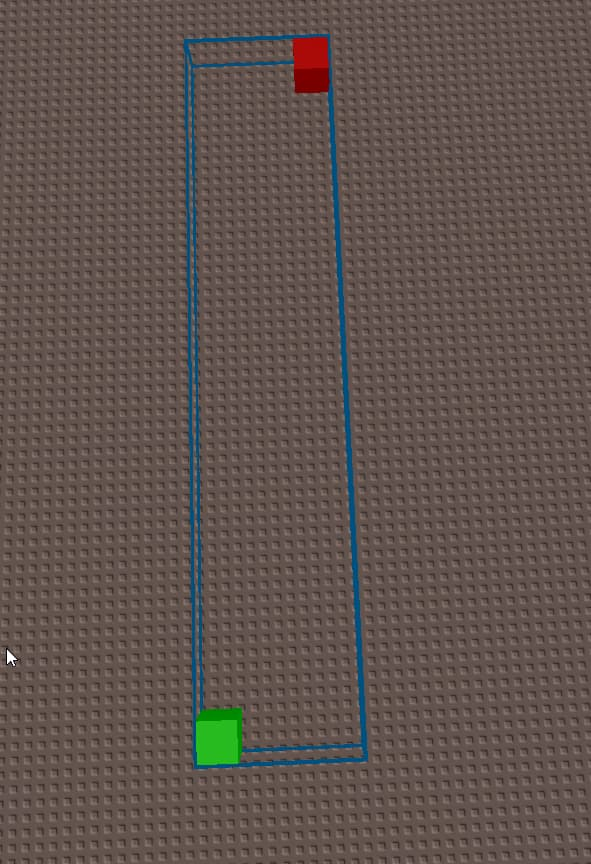# Place parts in bounding box

So this question may seem complex (I’m guessing?) as I haven’t gotten a fully working answer to this topic but I need to place parts in this bounding box but I don’t know how to programmatically.

Both parts take a value of 3 in size across all axis.

It should results into something like this (arrows defining the order)

If the parts are the same size in all 3 dimensions you could just treat them as voxels and for loop, their z coordinates through their x coordinates with the step being the size.

``````local part1 --defines the location of the box
local part2 --defines the end location of the box
for x=part1.X, part2.X, part1.Size.X do
for z=part1.Z,part2.Z,part1.Size.Z do
createNewPartAt(Vector3.new(x,part1.Y,z))
end
end
``````
2 Likes

Hey appreciate your response, but I’ve ran into an issue. It does work fine when the first point is collinear with the second point on the left side but breaks when the first point is shifted in different corners or when moved up on the y-axis. And I believe this doesn’t take in count of which point has the highest value to adjust to which may be why this isn’t fully working. I’ve attached visual reference and a studio file to test this.Game file : boundtest.rbxl (32.1 KB)

Try this

``````local part1 = workspace.Model.Part1
local part2 = workspace.Model.Part2

local function createNewPart(position)
local instance = Instance.new('Part')
instance.Name = 'Created'
instance.Size = Vector3.new(3, 3, 3)
instance.Position = position
instance.CFrame = instance.CFrame * CFrame.new(0,8,0)
instance.Anchored = true
instance.Color = Color3.new(0, 255, 0)
instance.Parent = workspace
end

local minX = math.min(part1.Position.X, part2.Position.X)
local maxX = math.max(part1.Position.X, part2.Position.X)
local minZ = math.min(part1.Position.Z, part2.Position.Z)
local maxZ = math.max(part1.Position.Z, part2.Position.Z)
local minY = math.min(part1.Position.Y, part2.Position.Y)
local maxY = math.max(part1.Position.Y, part2.Position.Y)
local origin = Vector2.new(part1.Position.X, part1.Position.Z)
local projectedVector = Vector2.new(part2.Position.X, part2.Position.Z)-origin

for x=minX, maxX, part1.Size.X do
for z=minZ,maxZ, part1.Size.X do
local point = Vector2.new(x,z) - origin
local t = point:Dot(projectedVector)/(projectedVector.Magnitude*projectedVector.Magnitude)
local y = part1.Position.Y+(part2.Position.Y-part1.Position.Y)*t
createNewPart(Vector3.new(x,y,z))
end
end
``````
1 Like

Appreciate this answer but it’s not really what I needed though it’ll be useful for me later on. I did get it to work by modifying it slightly like this

``````for x=minX, maxX, 3 do
for y = minY, maxY, 3 do
for z = minZ, maxZ, 3 do
createNewPart(Vector3.new(x,y,z))
end
end